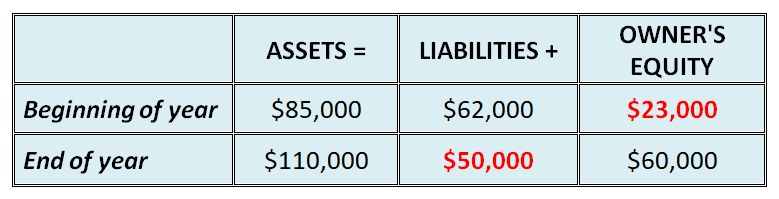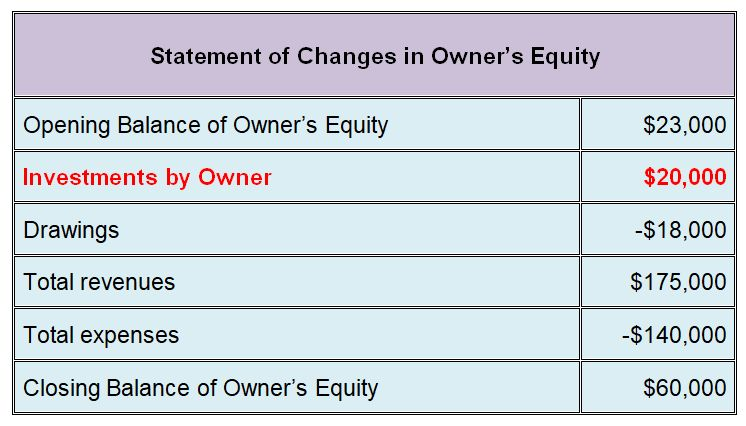# Accounting Equation Exercise:Fill in the Blank

by Sinthu

Before you begin: For purposes of testing and exams it's important to make sure you not only get the questions right but are completing them at the right speed. Use a watch or clock to time yourself for this exercise.

Difficulty Rating:
Beginner --> Intermediate

Time limit:
10 minutes

## Question:

The summaries of balance sheet and income statement data follow.

Beginning of year:
Total assets \$85,000
Total liabilities \$62,000
Total owner’s equity ?

End of year:
Total assets \$110,000
Total liabilities ?
Total owner’s equity \$60,000

Changes during year in owner’s equity:
Investments by owner ?
Drawings \$18,000
Total revenues \$175,000
Total expenses \$140,000

Required: Calculate the missing items above.

## Solution:

Beginning of year:

If Assets = Liabilities + Owners Equity
then
Owners Equity = Assets - Liabilities
= \$85,000 - \$62,000
=
\$23,000 Owners Equity

End of year:

If Assets = Liabilities + Owners Equity
then
Liabilities = Assets - Owners Equity
= \$110,000 - \$60,000
=
\$50,000 LiabilitiesChanges during the year in owner’s equity:

For this final part of the question we need to use the calculation of owner’s equity at the end of the year starting with equity at the beginning of the year plus/minus changes during the year
in order to work out the capital investment during the year. Or in simpler terms, reconstruct the statement of owner's equity .

Opening Balance \$23,000
Investments by owner ?
Drawings - \$18,000
Total revenues + \$175,000
Total expenses - \$140,000
Closing Balance \$60,000

To solve this problem we're going to take the statement of owner's equity with all the figures above and put them in a formula:

Closing Balance = Opening Balance + Investments by Owner - Drawings + Revenues - Expenses

Switching around this equation to make "Investments by Owner" (capital) the subject, we then get:

Investments by Owner = Closing Balance - Opening Balance + Drawings - Revenues + Expenses
= \$60,000 - \$23,000 + \$18,000 - \$175,000 + \$140,000
=
\$20,000 Investments by Owner

Here is how a complete statement of owner's equity would look:So, what did you think of this accounting equation exercise? Did you try it, and if so, how did you do? Leave us a comment further below.

If you had some difficulty with this exercise, don't worry! Check out some of the related tutorials and questions further below.

Best,
Michael Celender

Related Questions & Tutorials:

### Comments for Accounting Equation Exercise:Fill in the Blank

 Changes during the year in owner’s equity: by: Anonymous This is very confusing because the written formula you have does not match what's in the sample table. In the formula you have that you are adding +Drawings and in the table you have it(-) in red -18,000. This is very confusing to understand.Sorry that you're finding it confusing. The table is the normal calculation and format for the Statement of Changes in Owner's Equity, and the subject in that table is always the closing balance of owner's equity. I have shown the solution we obtained "Investments by Owner" (capital) in that table like it would normally appear if we were drawing up that statement. The written formula you're talking about (with + Drawings) is the switched-around version of the normal equity formula - we now have "Investments by Owner" (capital) as the subject instead of the closing balance of equity.In this question we know the closing balance of owner's equity and switch around the normal formula to get a new formula for "Investments by Owner" (capital).Maybe read the explanation one more time, hopefully it will make more sense.Best,Michael C.Founder of Accounting Basics for Students

Search this Site: